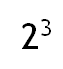Home Math Calculators Math  Flashcards Math Games Math  Homework Math Lessons Math Terms Math Worksheets Math Tools
You Are In Math Glossary of Terms

# Exponent

An exponent is a power that a number is raised to.

Example :

in,the exponent is 3.